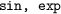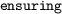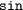## 1 Introduction

Numerical programs face an inherent tradeoff between accuracy and efficiency. Choosing a larger finite precision provides higher accuracy, but is generally more costly in terms of memory and running time. Not all applications, however, need a very high accuracy to work correctly. We would thus like to compute the results with only as much accuracy as is needed, in order to save resources.

Navigating this tradeoff between accuracy and efficiency is challenging. First, estimating the accuracy, i.e. bounding roundoff and approximation errors, is non-trivial due to the complex nature of finite-precision arithmetic which inevitably occurs in numerical programs. Second, the space of possible implementations is usually prohibitively large and thus cannot be explored manually.

Today, users can choose between different automated tools for analyzing accuracy of floating-point programs [7, 8, 11, 14, 18, 20, 26] as well as for choosing between different precisions [5, 6, 10]. The latter tools perform mixed-precision tuning, i.e. they assign different floating-point precisions to different operations, and can thus improve the performance w.r.t. a uniform precision implementation. The success of such an optimization is, however, limited to the case when uniform precision is just barely not enough to satisfy a given accuracy specification.

Another possible target for performance optimizations are elementary functions (e.g.). Users by default choose single- or double-precision libm library function implementations, which are fully specified in the C language standard (ISO/IEC 9899:2011) and provide high accuracy. Such implementations are, however, expensive. When high accuracy is not needed, we can save significant resources by replacing libm calls by coarser approximations, opening up a larger, and different tradeoff space than mixed-precision tuning. Unfortunately, existing automated approaches [1, 25] do not provide accuracy guarantees.

On the other hand, tools like Metalibm  approximate individual elementary functions by polynomials with rigorous accuracy guarantees given by the user. They, however, do not consider entire programs and leave the selection of its parameters to the user, limiting its usability mostly to experts.

We present an approach and a tool which leverages the existing whole-program error analysis of Daisy  and Metalibm’s elementary function approximation to provide both sound whole-program guarantees as well as efficient C implementations for floating-point programs with elementary function calls. Given a target error specification, our tool automatically distributes the error budget among uniform single or double precision arithmetic operations and elementary functions, and selects a suitable polynomial degree for their approximation.

We have implemented our approach inside the tool Daisy and compare the performance of generated programs against programs using libm on examples from literature. The benchmarks spend on average 38% and up to 50% of time for evaluation of the elementary functions. Our tool improves the overall performance by on average 14% and up to 25% when approximating each elementary function call individually, and on average 17% and up to 31% when approximating compound function calls. These improvements were achieved solely by optimizing approximations to elementary functions and illustrate pertinence of our approach. These performance improvements incur overall whole-program errors which are only 2–3 magnitudes larger than double-precision implementations using libm functions and are well below the errors of single-precision implementations. Our tool thus allows to effectively trade performance for larger, but guaranteed, error bounds.

Contributions. In summary, in this paper we present: (1) the first approximation technique for elementary functions with sound whole-program error guarantees, (2) an experimental evaluation on benchmarks from literature, and (3) an implementation, which is available at https://github.com/malyzajko/daisy.

Related Work. Several static analysis tools bound roundoff errors of floating-point computations [7, 18, 20, 26], assuming libm implementations, or verify the correctness of several functions in Intel’s libm library . Muller  provides a good overview of the approximation of elementary functions. Approaches for improving the performance of numerical programs include mixed-precision tuning [5, 6, 10, 16, 24], and autotuning, which performs low-level real-value semantics-preserving transformations [23, 27]. These leverage a different part of the tradeoff space than libm approximation and are thus orthogonal. Herbie  and Sardana  improve accuracy by rewriting the non-associative finite-precision arithmetic, which is complementary to our approach. Approaches which approximate entire numerical programs include MCMC search , enumerative program synthesis  and neural approximations . Accuracy is only checked on a small set of sample inputs and is thus not guaranteed.

## 2 Our Approach

We explain our approach using the following example  computing a forward kinematics equation and written in Daisy’s real-valued specification language:

Although this program is relatively simple, it still presents an opportunity for performance savings, especially when it is called often, e.g. during the motion of a robotics arm. Assuming double-precision floating-point arithmetic and library implementations for sine, Daisy’s static analysis determines the worst-case absolute roundoff error of the result to be 3.44e-15. This is clearly a much smaller error than what the user requested (1e-11) in the postcondition (clause).

The two elementary function calls toaccount for roughly 40.7% of the overall running time. We can save some of this running time using polynomial approximations, which our tool generates in less than 6 min. The new double precision C implementation is roughly 15.6% faster than one with libmFootnote 1 functions, i.e. using around 40% of the available margin. This is a noteworthy performance improvement, considering that we optimized uniquely the evaluation of elementary functions. The actual error of the approximate implementation is 1.56e-12, i.e. roughly three orders of magnitude higher than the libm error. This error is still much smaller than if we had used a uniform single precision implementation, which incurs a total error of 1.85e-6.

We implement our approach inside the Daisy framework , combining Daisy’s static dataflow analysis for bounding finite-precision roundoff errors, Metalibm’s automated generation of efficient polynomial approximations, as well as a novel error distribution algorithm. Our tool furthermore automatically selects a suitable polynomial degree for approximations to elementary functions.

Unlike previous work, our tool guarantees that the user-specified error is satisfied. It soundly distributes the overall error budget among arithmetic operations and libm calls using Daisy’s static analysis. Metalibm uses the state-of-the art minimax polynomial approximation algorithm  and Sollya  and Gappa  to bound errors of their implementations. Given a function, a target relative error bound and implementation parameters, Metalibm generates C code. Our tool does not guarantee to find the most efficient implementation; the search space of implementation and approximation choices is highly complex and discrete, and it is thus infeasible to find the optimal parameters.

The input to our tool is a straight-line programFootnote 2 with standard arithmetic operators ($$=, -, *, /$$) as well as the most commonly used elementary functions ($$\sin {}, \cos {}, \tan {}, \log {}, \exp {}, \sqrt{}$$). The user further specifies the domains of all inputs, together with a target overall absolute error which must be satisfied. The output is C code with arithmetic operations in uniform single or double precision, and libm approximations in double precision (Metalibm’s only supported precision).

Algorithm. We will use ‘program’ for the entire expression, and ‘function’ for individual elementary functions. Our approach works in the following steps.

Step 1 We re-use Daisy’s frontend which parses the input specification. We add a pre-processing step, which decomposes the abstract syntax tree (AST) of the program we want to approximate such that each elementary function call is assigned to a fresh local variable. This transformation eases the later replacement of the elementary functions with an approximation.

Step 2 We use Daisy’s roundoff error analysis on the entire program, assuming a libm implementation of elementary functions. This analysis computes a real-valued range and a worst-case absolute roundoff error bound for each subexpression in the AST, assuming uniform single or double precision as appropriate. We use this information in the next step to distribute the error and to determine the parameters for Metalibm for each function call.

Step 3 This is the core step, which calls Metalibm to generate a (piece-wise) polynomial approximation for each elementary function which was assigned to a local variable. Each call to Metalibm specifies the local target error for each function call, the polynomial degree and the domain of the function call arguments. To determine the argument domains, we use the range and error information obtained in the previous step. Our tool tries different polynomial degrees and selects the fastest implementation. We explain our error distribution and polynomial selection further below.

Metalibm generates efficient double-precision C code including argument reduction (if applicable), domain splitting, and polynomial approximation with a guaranteed error below the specified target error (or returns an error). Metalibm furthermore supports approximations with lookup tables, whose size the user can control manually via our tool frontend as well.

Step 4 Our tool performs roundoff error analysis again, this time taking into account the new approximations’ precise error bounds reported by Metalibm. Finally, Daisy generates C code for the program itself, as well as all necessary headers to link with the approximation generated by Metalibm.

Error Distribution. In order to call Metalibm, Daisy needs to determine the target error for each libm call. Recall that the user of our tool only specifies the total error at the end of the program. Hence, distributing the total error budget among arithmetic operations and (potentially several) elementary function calls is a crucial step. Consider again our running example which has two elementary function calls. Our tool distributes the error budget as follows:

\begin{aligned} | f(x) - \tilde{f}(\tilde{x}) | \le | f(x) - \hat{f}_1(x) | + | \hat{f}_1(x) - \hat{f}_2(x)| + | \hat{f}_2(x) - \tilde{f}(\tilde{x}) | \end{aligned}

where we denote by f the real-valued specification of the program; $$\hat{f}_1$$ and $$\hat{f}_2$$ have one and two elementary function calls approximated, respectively, and arithmetic is considered exact; and $$\tilde{f}$$ is the final finite-precision implementation.

Daisy first determines the budget for the finite-precision roundoff error ($$|\hat{f}_2(x) - \tilde{f}(\tilde{x})|$$) and then distributes the remaining part among libm calls. At this point, Daisy cannot compute $$|\hat{f}_2(x) - \tilde{f}(\tilde{x})|$$ exactly, as the approximations are not available yet. Instead, it assumes libm-based approximations as baseline.

Then, Daisy distributes the remaining error budget either equally among the elementary function calls, or by taking into account that the approximation errors are propagated differently through the program. This error propagation is estimated by computing the derivative w.r.t. to each elementary function call (which gives an estimation of the conditional number). Daisy computes partial derivatives symbolically and maximizes them over the specified input domain.

Finally, we obtain an error budget for each libm call, representing the total error due to the elementary function call at the end of the program. For calling Metalibm, however, we need the local error at the function call site. Due to error propagation, these two errors can differ significantly, and may lead to overall errors which exceed the error bound specified by the user. We estimate the error propagation using a linear approximation based on derivatives, and use this estimate to compute a local target error from the total error budget.

Since Metalibm usually generates approximations with slightly tighter error bounds than asked for, our tool performs a second roundoff analysis (step 4), where all errors (smaller or larger) are correctly taken into account.

Polynomial Degree Selection. The polynomial degree significantly and in a discrete way influences the efficiency of approximations, so that optimal prediction is infeasible. Hence, our tool performs a linear search, using the (coarse) estimated running time reported by Metalibm (obtained with a few benchmarking runs) to select the approximation with the smallest estimated running time. The search stops either when the estimated running time is significantly higher than the current best, or when Metalibm times out.

We do not automatically exploit other Metalibm’s parameters, such as minimum subdomain width for splitting, since they give fine-grained control that is not suitable for general automatic implementations.

## 3 Experimental Evaluation

We evaluate our approach in terms of accuracy and performance on benchmarks from literature [9, 19, 28] which include elementary function calls, and extend them with the examples rodriguesRotationFootnote 3 and ex2* and ex3_d, which are problems from a graduate analysis textbook. While they are relatively short, they represent important kernels usually employing several elementary function callsFootnote 4. We base target error bounds on roundoff errors of a libm implementation: middle and large errors, each of which is roughly three and four orders of magnitudes larger than the libm-based bound, respectively. By default, we assume double 64 bit precision.

Our tool provides an automatic generation of benchmarking code for each input program. Each benchmarking executable runs the Daisy-generated code on $$10^7$$ random inputs from the input domain and measures performance in the number of processor clock cycles. Of the measured number of cycles we discard the highest 10%, as we have observed these to be outliers.

Experimental Results. By default, we approximate individual elementary function calls separately, use equal error distribution and allow table-based approximations with an 8-bit table index. For large errors we also measure performance for: (i) default settings but with the derivative-based errors distribution; (ii) default settings but without table usage; (iii) default settings but with compound calls with depth 1 and depth $$\infty$$ (approximation ‘as much as possible’).

Table 1 shows the performance improvements of approximated code w.r.t. libm based implementations of our benchmarks. We compare against libm only, as no approximation or synthesis tool provides error guarantees. By removing libm calls in initial programs we roughly estimate the elementary function overhead (second column) and give an idea for the margin of improvement. Figure 1 illustrates the overall improvement that we obtain for each benchmark (the height of the bars) and the relative distribution of the running time between arithmetic (blue) and elementary functions (green), for large errors with default settings but approximate compound calls with depth = $$\infty$$.

Our tool generates code with significant performance improvements for most functions and often reduces the time spent for the evaluation of elementary functions by a factor of two. As expected, the improvements are overall better for larger errors and vary on average from 10.7% to 13.8% for individual calls depending on the settings, and reach 17.1% on average when approximating compound calls as much as possible. However, increasing the program target error (for equal error distributions Metalibm target error increases linearly with it) does not necessarily lead to better performance, e.g. in case of axisRotationY and rodriguesRotation. This is the result of discrete decisions concerning the approximation degrees and the domain splittings inside Metalibm.

Somewhat surprisingly, we did not observe an advantage of using the derivative-based error distribution over the equal one. We suspect that is due to the nonlinear nature of Metalibm’s heuristics.

Table 1 further demonstrates that usage of tables generally improves the performance. However, the influence of increasing the table size must be studied on a case-by-case basis since large tables might lead to memory-bound computations.

We observe that it is generally beneficial to approximate ‘as much as possible’. Indeed, the power of Metalibm lies in generating (piece-wise) polynomial approximations of compound expressions, whose behavior might be much simpler to evaluate than its individual subexpressions.

Finally, we also considered an implementation where all data and arithmetic operations are in single precision apart from the double-precision Metalibm-generated code (whose output is accurate only to single precision). We observe that slight performance improvements are possible, i.e. Metalibm can compete even with single-precision libm-based code, but to achieve performance improvements comparable to those of double-precision code, we need a single-precision code generation from Metalibm.

Analysis Time. Analysis time is highly dependent on the number of required approximations of elementary functions: each approximation requires a separate call to Metalibm whose running time in turn depends on the problem definition. Daisy reduces the number of calls to Metalibm by common expression elimination which improves the analysis time. Currently, we set the timeout for each Metalibm call to 3 min, which leads to an overall analysis time which is reasonable. Overall, our tool takes between 15 s and 20 min to approximate whole programs, with the average running time being 4 min 40 s per program.

## 4 Conclusion

We presented a fully automated approach which improves the performance of small numerical kernels at the expense of some accuracy by generating custom approximations of elementary functions. Our tool is parametrized by a user-given whole-program absolute error bound which is guaranteed to be satisfied by the generated code. Experiments illustrate that the tool efficiently uses the available margin for improvement and provides significant speedups for double-precision implementations. This work provides a solid foundation for future research in the areas of automatic approximations of single-precision and multivariate functions.